StatLect

# Factorization of joint probability mass functions

Given two discrete random variables (or random vectors)and, their joint probability mass function can be factorized into:

1. the conditional probability mass function ofgiven;

2. the marginal probability mass function of.## The factorization

The next proposition provides a formal statement of the factorization.

Proposition Letbe a discrete random vector with supportand joint probability mass function. Denote bythe conditional probability mass function ofgivenand bythe marginal probability mass function of. Then,for anyand.

Proof

See the lecture entitled Conditional probability distributions.

## A factorization method

If we need to derive the two factors from the joint probability mass function, we usually perform two steps:

1. marginalizeby summing it over all possible values ofand obtain the marginal probability mass function;

2. dividebyand obtain the conditional probability mass function(this can be done only if).

When the first step (marginalization) is too hard to perform, it is possible to avoid it thanks to a guess-and-verify procedure. The following proposition shows how.

Proposition Suppose there are two functionsandsuch that

1. for anyand, the following holds:2. for any fixed,, considered as a function of, is a probability mass function.

Then,Proof

We exploit the fact that the marginal probability mass function ofneeds to satisfyUsing this property in conjunction with property 1 in the proposition, we obtain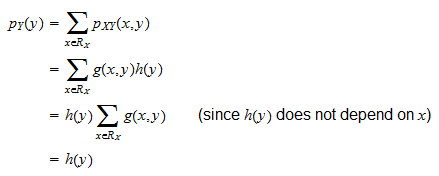The last equality is a consequence of the fact that, for any fixed,, considered as a function of, is a probability mass function and the sum of a probability mass function over its support equals. Thus,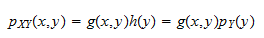Since we also have that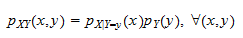then, by necessity, it must be thatThus, the guess-and verify procedure works as follows. First, we express the joint probability mass function as the product of two factors (this is the "guess" part). Then, we verify that:

1. one factor (a function ofand) is a probability mass function infor all values of;

2. the other factor (a function of) does not depend on.

Example Letbe arandom vector having a multinomial distribution with parameters,and(the probabilities of the three possible outcomes of each trial) and(the number of trials). The probabilities are strictly positive numbers such thatThe supportofis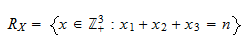The joint probability mass functionisWhen, we have that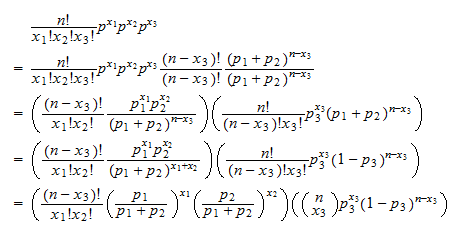whereis a binomial coefficient. Therefore, the joint probability mass function can be factorized aswhere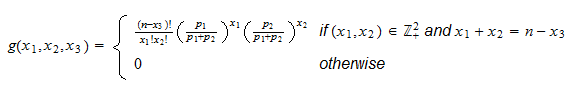and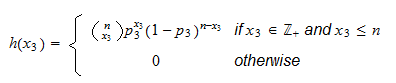But, for any,is the probability mass function of a multinomial distribution with parameters,and. Therefore,Note thatis the pmf of a binomial distribution with parametersand.